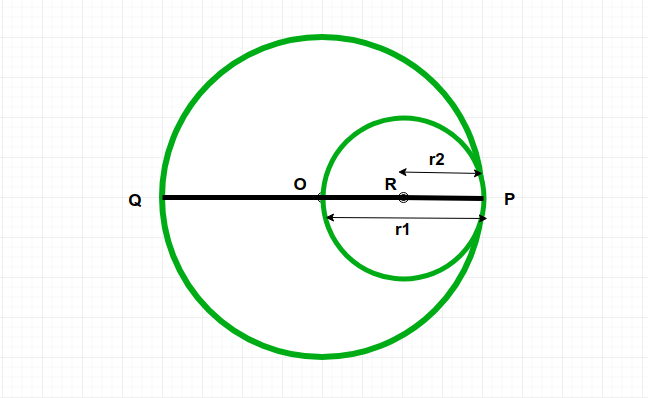# Program to calculate area of inner circle which passes through center of outer circle and touches its circumference

Given a circle C1 and it’s a radius r1. And one another circle C2 whose passes through center of circle C1 and touch the circumference of circle C1. The task is to find out the area of circle C2.
Examples:

Input: r1 = 4
Output:Area of circle c2 = 12.56

Input: r1 = 7
Output:Area of circle c2 = 38.465Approuch:
Radius r2 of circle C2 is.
So we know that the area of circle is.

Below is the implementation of the above approach:

## C++

 // C++ implementation of the above approach  #include   #include  using namespace std;     // Function calculate the area of the inner circle  double innerCirclearea(double radius)  {         // the radius cannot be negative      if (radius < 0)       {          return -1;      }         // area of the circle      double r = radius / 2;      double Area = (3.14 * pow(r, 2));         return Area;  }     // Driver Code  int main()  {             double radius = 4;      cout << ("Area of circle c2 = ",                  innerCirclearea(radius));      return 0;  }     // This code is contributed by jit_t.

## Java

 // Java implementation of the above approach     class GFG {         // Function calculate the area of the inner circle      static double innerCirclearea(double radius)      {             // the radius cannot be negative          if (radius < 0) {              return -1;          }             // area of the circle          double r = radius / 2;          double Area = (3.14 * Math.pow(r, 2));             return Area;      }         // Driver Code      public static void main(String arr[])      {          double radius = 4;          System.out.println("Area of circle c2 = "                            + innerCirclearea(radius));      }  }

## Python3

 # Python3 implementation of the above approach      # Function calculate the area of the inner circle   def innerCirclearea(radius) :         # the radius cannot be negative       if (radius < 0) :          return -1;                 # area of the circle      r = radius / 2;      Area = (3.14 * pow(r, 2));          return Area;          # Driver Code   if __name__ == "__main__" :             radius = 4;       print("Area of circle c2 =",              innerCirclearea(radius));      # This code is contributed by AnkitRai01

## C#

 // C# Implementation of the above approach  using System;         class GFG   {         // Function calculate the area       // of the inner circle      static double innerCirclearea(double radius)      {             // the radius cannot be negative          if (radius < 0)           {              return -1;          }             // area of the circle          double r = radius / 2;          double Area = (3.14 * Math.Pow(r, 2));             return Area;      }         // Driver Code      public static void Main(String []arr)      {          double radius = 4;          Console.WriteLine("Area of circle c2 = " +                             innerCirclearea(radius));      }  }      // This code is contributed by PrinciRaj1992

Output:

Area of circle c2 = 12.56


Attention reader! Don’t stop learning now. Get hold of all the important DSA concepts with the DSA Self Paced Course at a student-friendly price and become industry ready.

My Personal Notes arrow_drop_upCheck out this Author's contributed articles.

If you like GeeksforGeeks and would like to contribute, you can also write an article using contribute.geeksforgeeks.org or mail your article to contribute@geeksforgeeks.org. See your article appearing on the GeeksforGeeks main page and help other Geeks.

Please Improve this article if you find anything incorrect by clicking on the "Improve Article" button below.

Article Tags :
Practice Tags :

2

Please write to us at contribute@geeksforgeeks.org to report any issue with the above content.